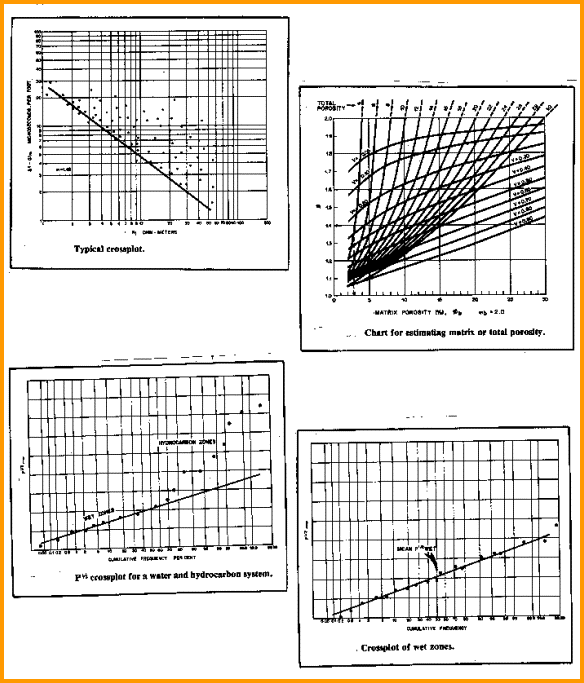Dual Porosity Model in Fractured Reservoirs
The first assumption made is that matrix and fractures are connected in parallel and 100 per cent saturated with formation water:

 Recall that we renamed the porosity terms as follpws:     5: PHIe = PHIm + PHIf     6: PHIt = PHIe + Vsh * BVWSH   Aquilera’s partitioning concept:     7: V = (PHIe - PHIm) / (PHIe * (1 - PHIm))

Since it is the breakdown of PHIe into PHIm and PHIf that we are seeking, equation 7 cannot help us find V directly. A useful approximation is:

 A practical partition factor     7a: V = (PHIe - PHIsc) / PHIe

This also assumes that PHIe >= PHIsc and that PHIsc is properly corrected for lithology and shale.

 Find Ro and Rfo     8: Ro = (1 / PHIe^Mb) * RW@FT     9: 1 / Rfo = V * PHIe / RW@FT + (1 - V) / Ro     10: F = Ro / RW@FT     11: Fd = Rfo / RW@FT   Combining equations:     12: Fd = 1 / (V * PHIe + (1 - V) / F)     13: PHIm = (((PHIe ^ Md) - (V * PHIe)) / (1 - V)) ^ (1/Mb)     14. PHIf = PHIe - PHIm

Where:
PHIe = effective porosity of dual porosity system (fractional), usually taken equal to PHIxnd from density neutron crossplot
PHIm = effective matrix porosity in dual porosity system (fractional)
PHIf = effective fracture porosity of dual porosity system (fractional)
PHIsc = sonic porosity (fractional)
Rfo = composite system resistivity at 100% Sw (ohm-m)
Ro = primary porosity resistivity at 100% Sw (ohm-m)
RW@FT = formation water resistivity at formation temperature (ohm-m)
F = formation factor for matrix rock (unitless)
Fd = formation factor for dual porosity model (unitless) Md = cementation exponent for dual porosity model, found from a Pickett plot
Mb = cementation exponent for un-fractured matrix rock, found from laboratory measurement, a Pickett plot in an un-fractured zone, or from assumption based on lithology
V = partitioning coefficient (fractional)Pickett plot, partition factor, and probability graphs for Aguilera’s dual porosity model

Top right shows the graphical solution to Equation 5 for various values of Mb. If, for example, Md is 1.4, Mb = 2.0, and effective porosity (PHIe) is 0.04, then the partitioning coefficient V is 0.26; thus, the fracture (-related) porosity (PHIf) is 0.0106 and the matrix porosity (PHIm) is 0.0294.

 The fracture porosity in this example is impossibly high. It must contain some vuggy or solution (fracture-related) porosity as well, so this method should be used with caution at all times.

To compare this result to core analysis, we must find matrix porosity from logs as a function of the matrix bulk volume (PHIcore), eliminating the volume of the fractures. Comparing to core porosity:
99: PHIcore = PHIm / (1 - PHIf)

Where:
PHIcore = effective matrix porosity corrected to match core (fractional)
PHIm = effective matrix porosity in double porosity system (fractional)
PHIf = effective secondary porosity of double porosity system (fractional)

For example, if V is 0.26 and PHIe is 0.04, then fracture porosity, PHIf, is 0.0106 and PHIm is 0.0294 as before, and:
PHIcore = 0.0294 / (1 - 0.0106) = 0.0297

NOTE: If the partitioning coefficient V cannot be found from sonic versus density neutron crossplot porosity, then equation 2 and 7 must be solved iteratively.

Fracture detection methods usually require that the fractures intersect the borehole and be invaded by mud of relatively low resistivity. This is not the case with the method presented here. In some cases it has been possible to detect fractures located within the radius of investigation of the logging tools, but not intercepted by the borehole.

RECOMMENDED PARAMETERS:
Md is in the range 1.0 to 1.8
Mb is in the range 1.6 to 2.6
V is in the range 0.0 to 0.5

Page Views ---- Since 01 Jan 2015
Copyright 2023 by Accessible Petrophysics Ltd.
CPH Logo, "CPH", "CPH Gold Member", "CPH Platinum Member", "Crain's Rules", "Meta/Log", "Computer-Ready-Math", "Petro/Fusion Scripts" are Trademarks of the Author